In the post Bitcoin trading with Python — Bollinger Bands strategy analysis the author reported 34% returns over the initial investment on back test. Does it work all the time? Is it the best strategy?

Let us use backtrader platform and compare several strategies just for buy signal. Backtrader is a feature-rich Python framework for backtesting and trading. Backtrader allows you to focus on writing reusable trading strategies, indicators and analyzers instead of having to spend time building infrastructure.

We will use the following strategies:

Crossover – based on two simple moving averages (SMA) with periods 10 and 30 days. Buy if fast SMA line crosses slow to the upside.

Consecutive 2 prices (Simple1) – Buy if two consecutive prices are decreasing – if current price is less than previous price and previous price is also less than previous price

  if self.dataclose < self.dataclose[-1]:
if self.dataclose[-1] < self.dataclose[-2]:


Consecutive 4 prices (Simple2) – Buy if within 4 price windows we have decrease more than 5% of original price between any two prices from this window:

   if tr_str == "simple2":
# since there is no order pending, are we in the market?
if not self.position: # not in the market
if (self.dataclose - self.dataclose[-1]) < -0.05*self.dataclose or (self.dataclose - self.dataclose[-2]) < -0.05*self.dataclose or (self.dataclose - self.dataclose[-3]) < -0.05*self.dataclose or (self.dataclose - self.dataclose[-4]) < -0.05*self.dataclose:
#if self.dataclose[-1] < self.dataclose[-2]:


Bollinger Bands – Buy when the price cross the bottom band.

  if self.data.close < self.boll.lines.bot:


Our starting asset value is 10000. We use daily prices.
After running above strategies we get results like below:Final Vaues for Strategies

cross 9999.06
simple1 9999.31
simple2 9999.91
BB 10011.099999999999


Thus we see that Bollinger Band looks more promising comparing with other strategies that we tried. However we did not do any optimization – using different parameters to improve performance of strategy. We learned how to set different strategies with backtrader and got understanding how to issue buy signal. Below you can find full source code.

# -*- coding: utf-8 -*-
import matplotlib
import matplotlib.pyplot as plt

from datetime import datetime

matplotlib.use('Qt5Agg')
plt.switch_backend('Qt5Agg')

# Create a subclass of Strategy to define the indicators and logic
class SmaCross(bt.Strategy):
# parameters which are configurable for the strategy
params = dict(
pfast=10,  # period for the fast moving average
pslow=30,   # period for the slow moving average
)
params['tr_strategy'] = None

def __init__(self):

self.boll = bt.indicators.BollingerBands(period=50, devfactor=2)
self.dataclose= self.datas.close    # Keep a reference to
self.sma1 = bt.ind.SMA(period=self.p.pfast)  # fast moving average
self.sma2 = bt.ind.SMA(period=self.p.pslow)  # slow moving average
self.crossover = bt.ind.CrossOver(self.sma1, self.sma2)  # crossover signal
self.tr_strategy = self.params.tr_strategy

def next(self, strategy_type=""):
tr_str = self.tr_strategy
print (self.tr_strategy)

# Log the closing prices of the series
self.log("Close, {0:8.2f} ".format(self.dataclose))
self.log('sma1, {0:8.2f}'.format(self.sma1))

if tr_str == "cross":
if not self.position:  # not in the market
if self.crossover > 0:  # if fast crosses slow to the upside

if tr_str == "simple1":

if not self.position: # not in the market
if self.dataclose < self.dataclose[-1]:
if self.dataclose[-1] < self.dataclose[-2]:

if tr_str == "simple2":

if not self.position: # not in the market
if (self.dataclose - self.dataclose[-1]) < -0.05*self.dataclose or (self.dataclose - self.dataclose[-2]) < -0.05*self.dataclose or (self.dataclose - self.dataclose[-3]) < -0.05*self.dataclose or (self.dataclose - self.dataclose[-4]) < -0.05*self.dataclose:

if tr_str == "BB":
#if self.data.close > self.boll.lines.top:
#self.sell(exectype=bt.Order.Stop, price=self.boll.lines.top, size=self.p.size)
if self.data.close < self.boll.lines.bot:

print('Current Portfolio Value: %.2f' % cerebro.broker.getvalue())

def log(self, txt, dt=None):
# Logging function for the strategy.  'txt' is the statement and 'dt' can be used to specify a specific datetime
dt = dt or self.datas.datetime.date(0)
print('{0},{1}'.format(dt.isoformat(),txt))

return

self.log('OPERATION PROFIT, GROSS {0:8.2f}, NET {1:8.2f}'.format(

strategy_final_values=[0,0,0,0]
strategies = ["cross", "simple1", "simple2", "BB"]

for tr_strategy in strategies:

cerebro = bt.Cerebro()  # create a "Cerebro" engine instance

data = bt.feeds.GenericCSVData(
dataname='GE.csv',

fromdate=datetime(2019, 1, 1),
todate=datetime(2019, 9, 13),

nullvalue=0.0,

dtformat=('%Y-%m-%d'),

datetime=0,
high=2,
low=3,
open=1,
close=4,
volume=6,
openinterest=-1

)

print ("data")
print (data )

# Print out the starting conditions
print('Starting Portfolio Value: %.2f' % cerebro.broker.getvalue())

result=cerebro.run()  # run it all
figure=cerebro.plot(iplot=False)
figure.savefig('example.png')

# Print out the final result
print('Final Portfolio Value: %.2f' % cerebro.broker.getvalue())
ind=strategies.index(tr_strategy)
strategy_final_values[ind] = cerebro.broker.getvalue()

print ("Final Vaues for Strategies")
for tr_strategy in strategies:
ind=strategies.index(tr_strategy)
print ("{} {}  ". format(tr_strategy, strategy_final_values[ind]))



## Python Files Tracker for Reducing Time Consuming Tasks

Do you want to know how many python files you create or update each year? Or do you need review actions to be completed next month?

Or you maybe run machine learning python models located in different folders, and find that it takes extra time to get back after switching priorities or working on different projects.

Here is the tool that can help with this – Python Files Tracker. The intent of this tool is eliminate time-consuming task of keeping track of python files with machine learning or other code. The tool allow to automate gathering and formatting information from notes saved in the comments.How it works.
You put some notes in python comments section created with triple quotation marks.

Then run python script and it will extract special notes plus file name, last modified date and save information in csv file. So you will have in one place all python file names and notes like what do next or what was wrong with the last run of machine learning model or what parameters were used.
The output information is saved in CSV file.

Very simple.

More Details on How to Use

The tool will extract notes that start from :. and end with .: and located within first python comment section. This section should start with triple quotation marks “”” and end also with quotation marks “””. Screenshot below demonstrates inserting specific notes for extraction by Files Tracker Tool: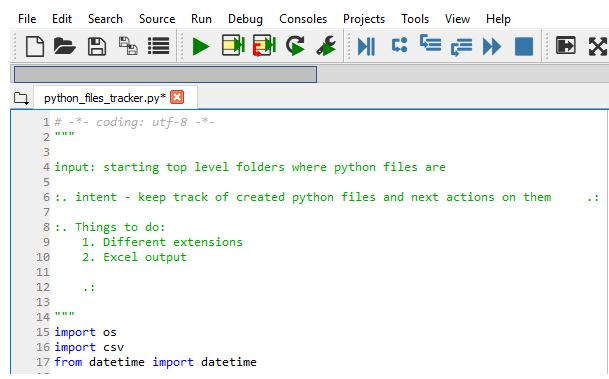Few special labels (intent, next action) can be inserted in the notes. The note with ‘intent’ label that is following after opening tag :. will be placed in the column Intent in CSV output file. And the note with ‘next action’ goes to Next action column.

If there is no label the text within :. and .: will be placed in Notes column in the output information.

The input to Python Files Tracker is folder or folders where python files are located. Only top level folder is required to specify. Python Files Tracker will look then in all sub folders. This is specified in the beginning of string in folders_top_level variable.

The tool is looking in all python files that have extension .py which is specified in variable ext. You can change extension and use the same for different files (for example php files). As long as the files are text files it will work.

Below is the source code for Python Files Tracker. Feel free to provide comments, feedback, request for adding different features. I would love to hear what do you think about the tool or how it works for you.

# -*- coding: utf-8 -*-
"""
Python Files Tracker

https://intelligentonlinetools.com/blog/2019/07/10/python-files-tracker/

Do not remove the this header
"""
import os
import csv
from datetime import datetime

ext=".py"
#INSERT here your own folders - you can have any number of folders separated by ; or just one folder.
folders_top_level="C:\\Users\\Desktop\\folder1;C:\\Users\\folder2"

def find_between( s, first, last ):

start_length=len(first)
try:
start = s.index( first ) + len( first )
end = s.index( last, start )
return s[start+start_length:end]
except ValueError:
return ""

def get_notes_from_file(filename):
tag_dictionary = {}
file_txt = open(filename, 'r', encoding="utf8")

start_pos=0
done=False
tag_dictionary['intent'] =""
tag_dictionary['next action'] =""
tag_dictionary['notes'] =""

while not done:

if (open_tag >= 0) :
else :

start_pos = open_tag+2
else:
done=True
return tag_dictionary

def create_dir(dirName):
if not os.path.exists(dirName):
os.mkdir(dirName)
print("Directory " , dirName ,  " Created ")
else:
print("Directory " , dirName ,  " already exists")

def get_file_contents(fname):

source_file = open(fname, 'r')
return source_code

def pywalker(path):

with open('data_files_tracker.csv', 'a', encoding="utf8", newline='' ) as csvfile:
writer = csv.DictWriter(csvfile, fieldnames=fieldnames)

print ("start")
for root, dirs, files in os.walk(path):

for dir_ in dirs:
print ( os.path.join(root, dir_) )

for file_ in files:

if file_.lower()[-len(ext):] == ext:
print( os.path.join(root, file_) )
full_fname=os.path.join(root, file_)
extracted_info=get_notes_from_file(full_fname)

print (extracted_info['intent'])

print (datetime.fromtimestamp(os.path.getmtime(os.path.join(root, file_))))

last_modified=datetime.fromtimestamp(os.path.getmtime(full_fname))

writer.writerow({'File': os.path.join(root, file_), 'Intent': extracted_info['intent'], 'Next action': extracted_info['next action'], 'Last modified':last_modified, 'Notes': extracted_info['notes'] })

if __name__ == '__main__':

folders= folders_top_level.split(";")
for folder in folders:
pywalker(folder)


References
1. Python 101 how to traverse a directory/

## Reinforcement Learning Python DQN Application for Resource Allocation

In the previous post Reinforcement Learning Example for Planning Tasks Using Q Learning and Dyna-Q we applied Dyna-Q algorithm for planning of actions to complete tasks. This problem can be viewed as resource allocation task. In this post we will use reinforcement learning python DQN (Deep Q-network) for the same problem. In case you did not read previous post the problem is described below.

## The Problem

Given some goals (projects to complete) and set of actions (number of hours to put for each project per day) we are interesting to know what action we need to take (how many hours to put per project on each day) in order to get the best result in the end (we have reward for completion project in time).

So we are trying to allocate resource (time) for each project for each day in such way that it produces maximum reward in the end of given period. We have reward data and time needed to complete for each project.

The diagram of one of possible path would look like this:

On this diagram the green indicates path that produces the max reward 13 as the agent was able to complete both goals.

## Deep Q-Network

Deep Q-Networks, abbreviated DQN, use deep neural networks as function approximation of the
action-value function q(s, a). The input of the artificial neural network used is the state and the output is the estimated q-values of the state-action pairs.

In DQN the replay memory simply stores the transitions such that they can be used at later times. By sampling transitions from the replay memory the network increases its ability to generalize. This also allows the network to predict the correct values in states which might be visited less frequently when the agent’s strategy gets better.

Also we add a second network, a target network, which is a copy of the first network, which we call the training network. The target network is only used to predict the value of taking the optimal action from s0 when updating the training network. The target network is updated with a certain frequency by copying the weights from the training network. This prevents instability when s and s0 are equal or even similar which is often the case. 

## Solution

The code here is based on DQN with Tensorflow for maze problem and previous code for Dyna-Q mentioned in the beginning of the post. It has 2 modules for programming environment and Reinforcement Learning Tensorflow DQN algorithm. Additionally it has main module which run the loop with episodes.

To run this reinforcement learning example you can use reinforcement learning python source code from the links below:

## Results

Below are charts obtained from running program. Performance (achieving max possible reward) with DQN is a little higher (but not significantly) than with Dyna-Q example on the same problem.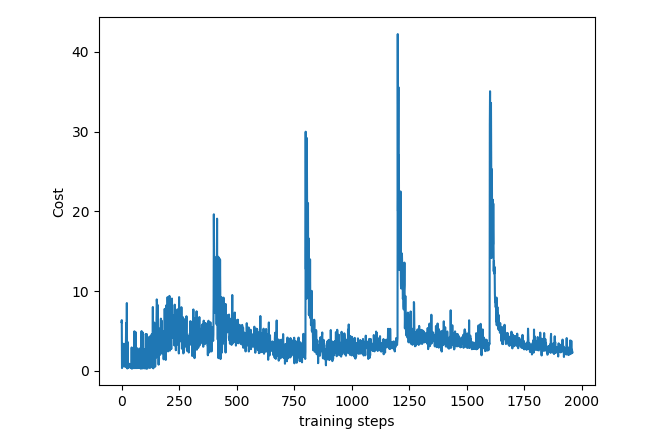References

1. Reinforcement learning for planning of a simulated production line Gustaf Ehn, Hugo Werner, February 27, 2018

## Application of Daubechies Wavelet for Denoising 1D Data

If we see in the real world, we will always face the signals which are not changing their stats. Means the change in the data signals are quite slow. But if we compare the 1D- data to the 2D Image data then we can see the 2D images have more drastic change in the magnitude of the pixels due to edges, change in the contrast and the two different things in the same image.

## Fourier Transform isn’t Able to Represent the Abrupt Changes Efficiently

So 1D data have slow oscillation but the images have more abrupt changes. These abrupt changing parts are always the interesting for that data as well as the images. They always show more relevant information for the images and the data.

Now, we have great tool for the analysis of the signals and that is the Fourier transform. But, it doesn’t able to represent the abrupt changes efficiently. That’s the demerit of the Fourier transform. The reason for this is that the Fourier transform is made up from the summation of the weighted sin and cosine signals. So, for abrupt changes that transform is less efficient.

## Wavelets and Wavelet Transform is Great Tool for Abrupt Data Analysis

For that problem we must find out different bases except the sin and cosine because these bases are not efficient for the abrupt representation. For the solution of these problems, another great tool came and those are the Wavelets and Wavelet transform. A wavelet is the rapidly decaying, wave like oscillation and that is also for the finite duration not like the sin and cosine (They oscillates forever.)

There are number of wavelets and based on the application and on the nature of the data, we can select the wavelet for that application and the data. Here, I have shown some of the well-known types of wavelets.Figure 1. Well known types of wavelets (Image is from MathWorks)

Now we are going to plot the Morlet in the MATLAB and that is quite easy if you know the basics of the MATLAB.

The equation for the Morlet wavelet is,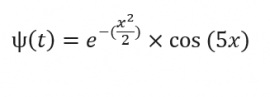Let us plot the Morlet function using MATLAB.

%% Morlet Wavelet functions
lb = -4;% lower bound
ub = 4;% uper bound
n = 1000; % number of points
x = linspace(lb,ub,n);
y = exp(((-1)*(x.^2))./2).*cos(5*x);
figure,plot(x,y,'LineWidth',2)
% title(['Morlet Wavelet']);
title('Morlet Wavelet $$\psi(t) = e^{\frac{-x^2}{2}} \cos(5x)$$','interpreter','latex')


If we plot this wave then we will get the result like below,Figure 2. Plot of the Morlet in the MATLAB.

We can see that this Morlet can able to represent the drastic changes and we can scale it for more drastic changes like Figure 3(b).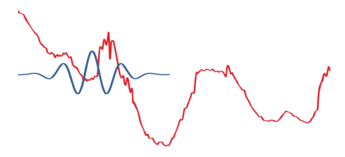Figure 3a: Less abrupt change and the signal is applied as it is.

Figure 3b: More abrupt change than the figure 3a. and in this case the signal is applied after some scaling.Figure 3c: More abrupt change than the figure 3b. and in this case the signal is applied after very high scaling to represent the very sharp abrupt change.

Now, we have understood what exactly the Wavelets are. These wavelets are the bases for the Wavelet Transform similar like Sine and Cosines are the bases for the Fourier Transform.

## The Wavelet Transform

The wavelet transform is the mathematical tool that can able to decomposes a signal into a representation of the signal’s fine details and the trends as the function of time. We can use this transform or this representation to characterize the abrupt changes or transient events, to denoise, to perform many more operations on that.

The main benefit of wavelet transform or methods over traditional Fourier transform or methods are the uses of localized basis functions called as the wavelets and it give more faster computation. Wavelets as being localized basis functions are best for analyzing real physical situations in which a signal have discontinuities, abrupt changes and sharp spikes.

Two major transforms that are very useful to wavelet analysis are the
Continuous Wavelet Transform
Discrete Wavelet Transform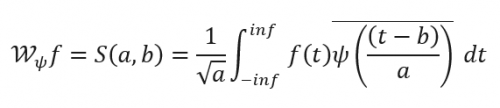If we see this equation then we will get feel like, oh!! That is very similar to the Fourier transform. Yes, that is very similar to that but here major difference is that ψ(t) and that is the wavelet not the sin and the cosine. Here as a ψ(t), we can take any wavelet that suit best for our applications. Now we will be going to discuss about the uses of the wavelet transform.

The following are applications of wavelet transforms:
Data and image compression
Transient detection
Pattern recognition
Texture analysis
Noise/trend reduction

## Wavelet Denoising

In this article we will go through the one application of the wavelet transform and that is denoising of 1-D data.
1-D Data:
I have taken the electrical data through the MATLAB.
I have taken only the some part of that signal for the process.
s = leleccum(1:3920);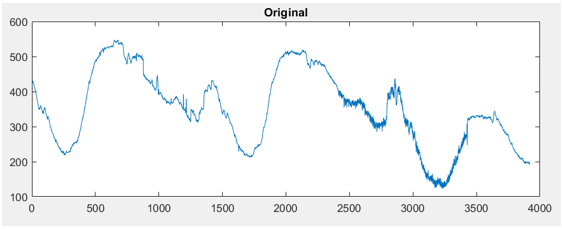Figure 4. Electrical Signal Lelecum from the MATLAB.

This signal have so much sharp and abrupt changes and we can see some additional noise as well from 2500 to 3500. Here we can use the wavelet transform to denoise this signal.

First, we will perform only the one step Wavelet Decomposition of a Signal. For one step we will get only the two components and one will be approximation and the second will be the detail of the signal. Here I have used the Daubechies wavelet for the wavelet transform.
[cA1,cD1] = dwt(s,’db1′);

This generates the coefficients of the level 1 approximation (cA1) and detail (cD1). This both are coefficients now we can construct the level 1 approximation and the detail as well.

A1 = upcoef('a',cA1,'db1',1,ls);
D1 = upcoef('d',cD1,'db1',1,ls);


If we display it then it will look something like Figure 5. We can see the approximation which are more and less similar to the signal and the details shows the sharp fluctuations of the signal.

Now, we will perform the decomposition of the signal in 3 levels. This decomposition will be the similar to the Figure 6. We can decompose the signal in these levels for more levels of details. Here we will get three level details cD1, cD2 and cD3 and one approximation cA3.

We can create this 3 level decomposition using the “wavedec” function from the MATLAB. This function used for the decomposition of the signal in to multi-level wavelet decomposition.
[C,L] = wavedec(s,3,’db1′);

Here also I have used the Daubechies wavelet. The coefficients of all the components of a third-level decomposition (that is, the third-level approximation and the first three levels of detail) are returned concatenated into one vector, C. Vector L gives the lengths of each component.Figure 5. Approximation A1 and detail D1 at the first step.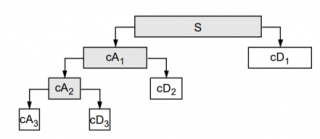Figure 6. Approximation and the details of the signal till level 3 (Image is from the MATHWORK).
We can extract the level 3 approximation coefficients from C using the “appcoef” function from the MATLAB.

cA3 = appcoef(C,L,'db1',3);


We can extract the level 3 details coefficients from C and L using the “detcoef” function from the MATLAB.

cD3 = detcoef(C,L,3);
cD2 = detcoef(C,L,2);
cD1 = detcoef(C,L,1);


This way we have total three values cA3, cD1, cD2, and cD3. We can reconstruct the approximate and details signals from these coefficients using “wrcoef”.

% To reconstruct the level 3 approximation from C,
A3 = wrcoef('a',C,L,'db1',3);

% To reconstruct the details at levels 1, 2 and 3,
D1 = wrcoef('d',C,L,'db1',1);
D2 = wrcoef('d',C,L,'db1',2);
D3 = wrcoef('d',C,L,'db1',3);


If we display this images then it will look something like Figure 7.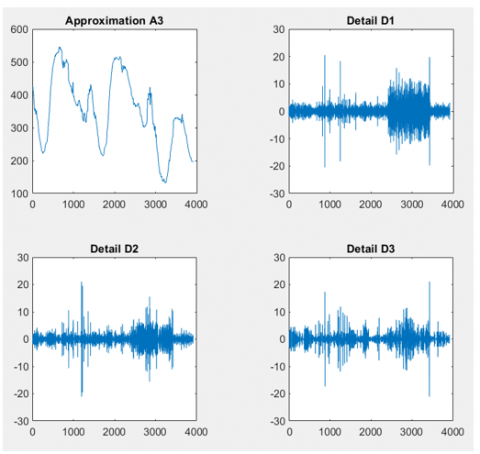Figure 7. Approximation and details at the different levels.

We can use the wavelets to remove noise from a signal but it will requires identifying which component or components have the noise and then recovering the signal without those components. In this example, we have observed that as we increase the number of the steps, the successive approximations become much less and less noisy because more and more high-frequency information is filtered out of the signal.

If we compare the level 3 approximation with the original signal then we can find that level 3 approximation is much more smother than the original signal.

Of course, after removing all the high-frequency information, we will have lost many abrupt information from the original signal. So for optimal de-noising will required a more subtle method and that is called as thresholding. Thresholding involves removing the portion from the details which have higher activity than the certain limits.

What if we limited the strength of the details by restricting their maximum values? This would have the effect of cutting back the noise while leaving the details unaffected through most of their durations. But there’s a better way. We could directly manipulate each vector, setting each element to some fraction of the vectors’ peak or average value. Then we could reconstruct new detail signals D1, D2, and D3 from the thresholded coefficients.

To denoise the image,

[thr,sorh,keepapp] = ddencmp('den','wv',s);
clean = wdencmp('gbl',C,L,'db1',3,thr,sorh,keepapp);


“ddencmp” function gives the default values of the threshold, SORH and KEEPAPP which allows you to keep approximation coefficients. Clean is the denoised signal.

Figure 8. Shows both the original as well as the clean signal.Figure 8. Original signal with the De-noised signal.

## Conclusion:

Wavelet are the great tools for the analysis of the signals and those signals have ability to representation the signal in the great detail. Here we have experimented with the denoising of the electrical signal, we have seen that using only low pass filter may affect the abrupt information of signals. But using the proper process of the wavelet transform we can have great denoised signal.

Wavelets can do much more than the denoising. Popular “.JPEG” encoding format for the images uses the discrete cosine transform for the compression of the images. There is other algorithm JPEG2000 which have great accuracy of the image with great compression. And JPEG2000 algorithm uses the wavelet transform.

Thus wavelets are very useful, so have great time with number of wavelets and may this article helps you to for the understanding of the wavelets.
For whole code in MATLAB and for more exciting projects please visit github.com/MachineLearning-Nerd/Wavelet-Analysis GITHUB repository.

## Integrating Sentiment Analysis API Python Django into Web Application

In this post we will learn how to use sentiment analysis with API python from paralleldots.com. We will look at running this API from python environment on laptop and also in web application environment with python Django on pythonanywhere hosting site.

In the one of previous post we set python Django project for chatbot. Here we will add file to this environment. Setting the chatbot files from previous project is not necessary. We just need folder structure.

Thus in this post we will reuse and extend some python Django knowledge that we got in the previous post. We will learn how to pass parameters from user form to server and back to user form, how to serve images, how to have logic block in web template.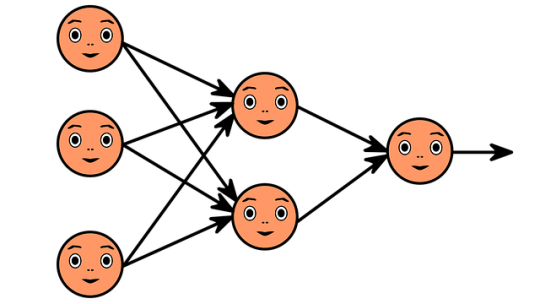ParrallelDots  provides several machine learning APIs such as named entity recognition (NER), intent identification, text classification and sentiment analysis. In this post we will explore sentiment analysis API and how it can be deployed on web server with Diango python.

## Running Text Analysis API Locally

First we need install the library:
pip install paralleldots

We need also obtain key. It is free and no credit card required.

Now we run code as below

import paralleldots
paralleldots.set_api_key("XXXXXXXXXXX")

# for single sentence
text="the day is very nice"
lang_code="en"
response=paralleldots.sentiment(text,lang_code)

print(response)
print (response['sentiment'])
print (response['code'])
print (response['probabilities']['positive'])
print (response['probabilities']['negative'])
print (response['probabilities']['neutral'])

# for multiple sentence as array
text=["the day is very nice,the day is very good,this is the best day"]
response=paralleldots.batch_sentiment(text)
print(response)

Output:
{'probabilities': {'negative': 0.001, 'neutral': 0.002, 'positive': 0.997}, 'sentiment': 'positive', 'code': 200}
positive
200
0.997
0.001
0.002
{'sentiment': [{'negative': 0.0, 'neutral': 0.001, 'positive': 0.999}], 'code': 200}


This is very simple. Now we will deploy on web hosting site with python Django.

## Deploying API on Web Hosting Site

Here we will build web form. Using this web form user can enter some text which will be passed to semantic analysis API. The result of analysis will be passed back to user and image will be shown based on result of sentiment analysis.
First we need install paralleldots library. To install the paralleldots module for Python 3.6, we’d run this in a Bash console (not in a Python one): 
pip3.6 install –user paralleldots

Note it is two dashes before user.
Now create or update the following files:

views.py

In this file we are getting user input from web form and sending it to API. Based on sentiment output from API we select image filename.

from django.shortcuts import render

import paralleldots

def do_sentiment_analysis(request):
user_sent=""
user_input=""
fname="na"
if request.POST:
user_input=request.POST.get('user_input', '')
lang_code="en"
paralleldots.set_api_key("XXXXXXXXX")
user_response=paralleldots.sentiment(user_input,lang_code)
user_sent=user_response['sentiment']

if (user_sent == 'neutral'):
fname=  "emoticon-1634586_640.png"
elif (user_sent == 'negative'):
fname = "emoticon-1634515_640.png"
elif (user_sent == 'positive'):
fname = "smiley-163510_640.jpg"
else:
fname="na"

return render(request, 'my_template_img.html', {'resp': user_sent, 'fname':fname, 'user_input':user_input})


my_template_img.html
Create new file my_template_img.html This file will have web input form for user to enter some text. We have also if statement here because we do not want display image when the form is just opened and no submission is done.

<html>
<form method="post">
{% csrf_token %}

<textarea rows=10 cols=50 name="user_input">{{user_input}}</textarea>
<br>
<label>{{resp}}</label>
<br>
<button type="submit">Submit</button>

{% if "_640" in fname %}
<img src="/media/{{fname}}" width="140px" height="100px">
{% endif %}
</form>
</html>


Media folder
In the media folder download images to represent negative, neutral and positive. We can find images on pixabay site.

So the folder can look like this. Note if we use different file names we will need adjust the code.

 emoticon-1634515_640.png
emoticon-1634586_640.png
smiley-163510_640.jpg



urls.py
This file is located under /home/username/projectname/projectname. Add import line to this file and also include pattern for do_sentiment_analysis:

from views import do_sentiment_analysis

urlpatterns = [

url(r'^press_my_buttons/$', press_my_buttons), url(r'^do_sentiment_analysis/$', do_sentiment_analysis),

]


settings.py

This file is also located under /home/username/projectname/projectname
Make sure it has the following

STATIC_URL = '/static/'

MEDIA_URL = '/media/'

STATIC_URL = '/static/'


Now when all is set, just access link. In case we use pythonanywhere it will be: http://username.pythonanywhere.com/do_sentiment_analysis/

Enter some text into text box and click Submit. We will see the output of API for sentiment analysis result and image based on this sentiment. Below are some screenshots.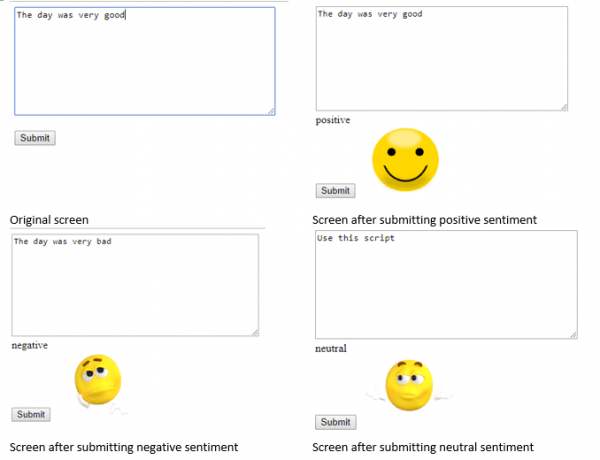Conclusion
We integrated machine learning sentiment analysis API from parallelDots into our python Diango web environment. We built web user input form that can send data to this API and receive output from API to show it to user. While building this we learned some Django things:
how to pass parameters from user form to server and back to user form,
how to serve images,
how to have logic block in web template.
We can build now different web applications that would use API service from ParallelDots. And we are able now integrate emotion analysis from text into our website.

References